# Classification of medical device using different Machine Learning techniques

## Autor: Agustín Bignú

Physicist – Machine Learning engineer.

### Abstract

In this paper, a classification was made of three classes of medical devices manufactured by Stening®. The classification was made using three machine learning algorithms. These algorithms were Support Vector Machines, Logistic Regression and Decision Tree. With this study Stening® aims to make their products more known and that one can have a more technical vision of these.

Kew words: Machine Learning. Silicone stent. Tracheal prostheses. Support Vector Machine. Logistic Regression. Decision Tree.

### Introduction

The main objectives of this study are:

• publicize the products that Stening® manufactures
• offer a more technical view on these using innovative technology

In this paper, a classification of three classes of medical devices manufactured by Stening® was made. For this, three different machine learning classification techniques were used. The three algorithms do the same but their internal functioning is totally different. These were: Support Vector Machines (SVM), Logistic Regression (LG) and Decision Tree (DT). The internal operation will be explained in the theoretical basis.
The medical devices that were classified were the following:

• ST (Tracheal stent)
• TM (T tube)
• SY (“Y” stent)

Three datasets of 900, 1200 and 1500 rows were generated to carry out the training. For the classification we rely on 5 different but common attributes of the devices: length, diameter, anchors, number of branches and width of the wall. These 5 attributes are what differentiate the devices. The creation of the dataset will be explained in the result section.

We will analyze the results of each algorithm applied to the three datasets, this will be done in the result section.

Finally, the conclusions and future steps of this study will be given.

### Kind of study

Computational study oriented to the artificial intelligence sector.

### Theoretical basis

In this section we will introduce the algorithms used for the classification of medical devices.

#### SVM

In this section, the Support Vector Machines algorithm will be introduced . It is a classification algorithm that belongs to the branch of supervised learning. The classification is done by finding the best hyperplane1 in an N-dimensional space (N is the number of parameters) that separates the data.

There are many possible hyperplanes to separate two kinds of data. The objective of the algorithm is to find the one with the greatest distance between the data of both classes (see figure 1).

The hyperplanes delimitate the area where one class begins and another ends. That is, if in the future we receive a parameter in the lower area of the hyperplane it will be of the red class; if it is in the other zone it will be blue. The hyperplanes can be of different dimensions, it depends on the data we have. If we have a data set {x1, x2}, as in Figure 1, our hyperplane will be a line, and if the data depends on three different attributes: {x1, x2, x3} the hyperplane will be of dimension two. In other words, it will always have one less dimension than our data set.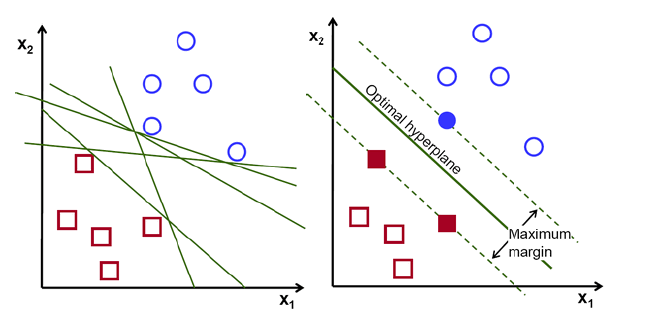Figure 1: Representation of hyperplanes in two dimensions .

The support vectors are data that are close to the hyperplane and influence its orientation and positioning. In SVM we seek to maximize the margin between the points and the hyperplane.

The function that helps us maximize the margin is the following: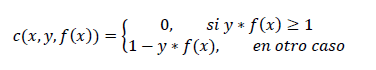(1)
The expression (1) is called the loss function. In this expression, x is the data, y it is the known result and f(x) is the prediction we make. If these last two are of the same sign, the loss function is zero.

This algorithm is used to perform classification problems and to make predictions. For example, distinguishes between three types of flowers from three different attributes: x = {color, width of the petals, height}. Then, by learning from the data, the model must be able to predict what kind of flower (y) belongs a flower with attributes it has not seen.

#### Logistic Regression

Logistic regression is a statistical method to perform classifications . It is a special type of linear regression where the classes to predict are categorical. This can be understood with an example: predict whether I have an illness or not, the answers would be ‘Yes’ or ‘No’.

As it was said is a special type of linear regression. The formula for a linear regression is as follows:(2)
In the expression (2) y is the result of the prediction and X1, X2, … are the variables with which the model trains. We obtain the logistic regression by introducing (2) in the following expression: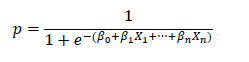(3)
The expression (3) is called the Sigmoid function (Figure 2). Then, the logistic regression consists of applying the sigmoid function to a linear regression.

We have three types of logistic regressions:

• Binary logistic regression: two categories to predict
• Multinomial logistic regression: three or more categories to predict
• Ordinal logistic regression: three or more categories to predict but with a certain order

In this paper we will use multinomial logistic regression since we have three kind of medical devices, it will not be ordinal because they lack order.Figure 2: Graphical representation of a binary logistic regression .

#### Decision Tree

A decision tree is a widely used algorithm, not only in Machine Learning but in other areas of computing . Its logic is simpler than that of the other two algorithms because it is more intuitive.

The decision tree has three main components: nodes, leaves and branches. A node represents an attribute, a branch represents a decision and a leaf represents an output. The main objective is to generate a decision tree that has as many leafs as categories you want to classify, in our case three since we have three different types of medical device. The structure would look like the one in figure 3.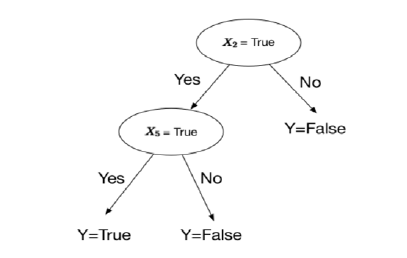Figure 3: Graphic representation of a decision tree .

### Results

In this section we will explain how we generated the dataset and the results obtained.

#### Dataset

The dataset was made from the measurements of the original Stening® devices. Three different datasets were made of 900, 1200 and 1500 rows each.

As it was said the datasets were generated randomly from the dimensions of the devices manufactured by Stening®. This gave us a freedom of training by having a greater variety. This is because some dimensions that are included in Stening®‘s website are not the most sold and therefore the least manufactured. So if we rely on that to generate the dataset it would be biasing the model and training. That is why, as a first approximation, we decided to generate the dataset this way. However, as said, always within the real dimensions of the devices.

In the following image we can see a few rows of one of the datasets: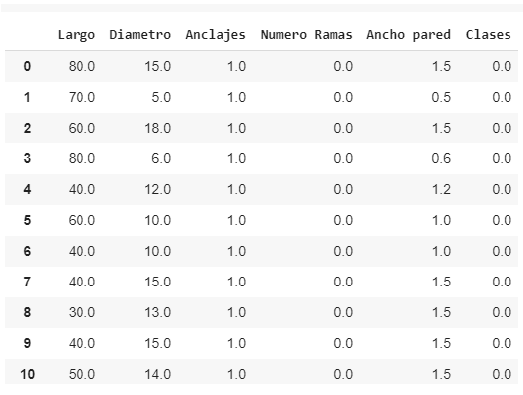Figure 4: First 11 rows of the 1200-row dataset.

Being 3 different kind of devices, they were assigned with a number to train the models. In this way: ‘0’ represents an ST, ‘1’ a TM and ‘2’ an SY. In turn, for the binary parameters (Yes / No) such as the column (“Anchors”), a ‘1’ was assigned if the answer was ‘Yes’ and a ‘0’ if the answer was ‘No’.

A program written in Python 3.5 was used to generate the dataset.

#### Programs and results

Three programs were carried out, one for each model to be applied. The inputs of the three programs were the three datasets. Obtaining thus, three results per algorithm. These three programs were written, like the one generated by the dataset, in Python 3.5. To make them, the Sklearn machine learning library was used.

As for the results, absolute efficiency was obtained in the three models. Each of them was able to obtain 100% efficiency in predicting samples that they had not seen before. In the following images we can see the results: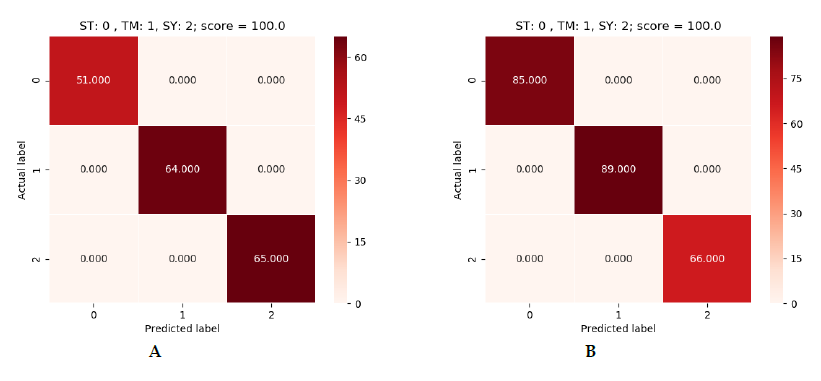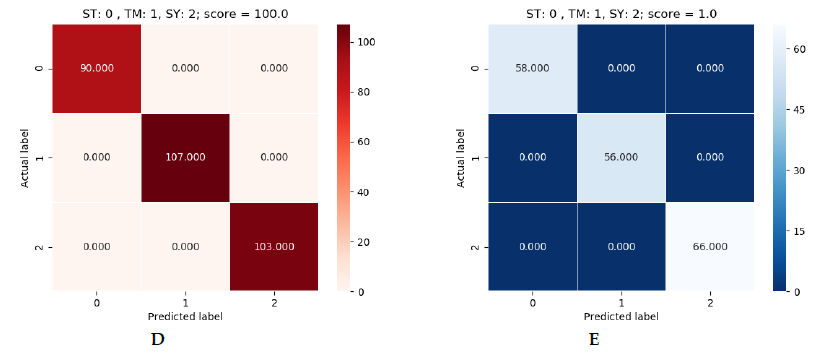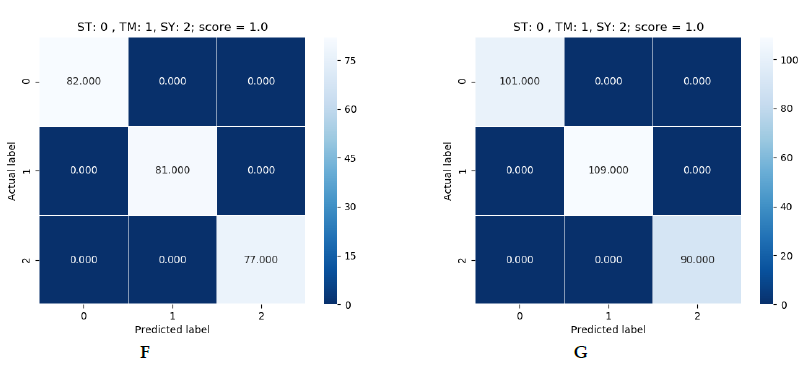Figure 5: green (SVM), blue (Logistic Regression) and red (Decision Tree).

In Figure 5 we can see the results for each data set. As said, there are three boards per algorithm. They are in order, the first being the 900 and the last the 1500. In each table we can see a reference to the labels. On the vertical axis we have the correct label and on the horizontal axis the label predicted by the model. In the upper right corner we have the results of the prediction. The number that appears inside the square is the number of devices predicted with those labels. If we add all the numbers we will see that we will not obtain the total of the dataset since these results are the predictions on a part of the dataset that the model did not see during the training. This is because the dataset was divided into 70% for training and 30% for testing.

### Conclusions

To conclude, it is worth mentioning that the results obtained are very satisfactory. This motivates us to continue researching and carrying out studies related to machine learning and artificial intelligence.
In future studies, improvements will be made such as training with real devices in market stock as well as applying similar machine learning techniques to other branches of Stening® devices.

### Footnotes

1. It is a plane of dimension N-1, where N is the total dimension of the space in which we are (1D, 2D, 3D …)

### References

1. Support Vector Machine, https://towardsdatascience.com/support-vector-machine-introduction-to-machine-learning-algorithms-934a444fca47
2. Support Vector Machine, https://towardsdatascience.com/ support-vector-machine-introduction-to-machine-learning-algorithms-934a444fca47 Fig. 1
3. Logistic Regression, https://www.datacamp.com/community/tutorials/understanding-logistic-regression-python
4. Decision Tree, https://medium.com/deep-math-machine-learning-ai/chapter-4-decision-trees-algorithms-b93975f7a1f1
5. Decision Tree, https://hackernoon.com/what-is-a-decision-tree-in-machine-learning-15ce51dc445d.

Original paper